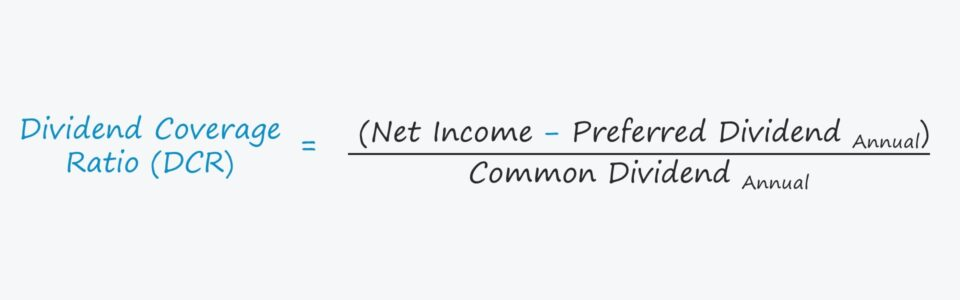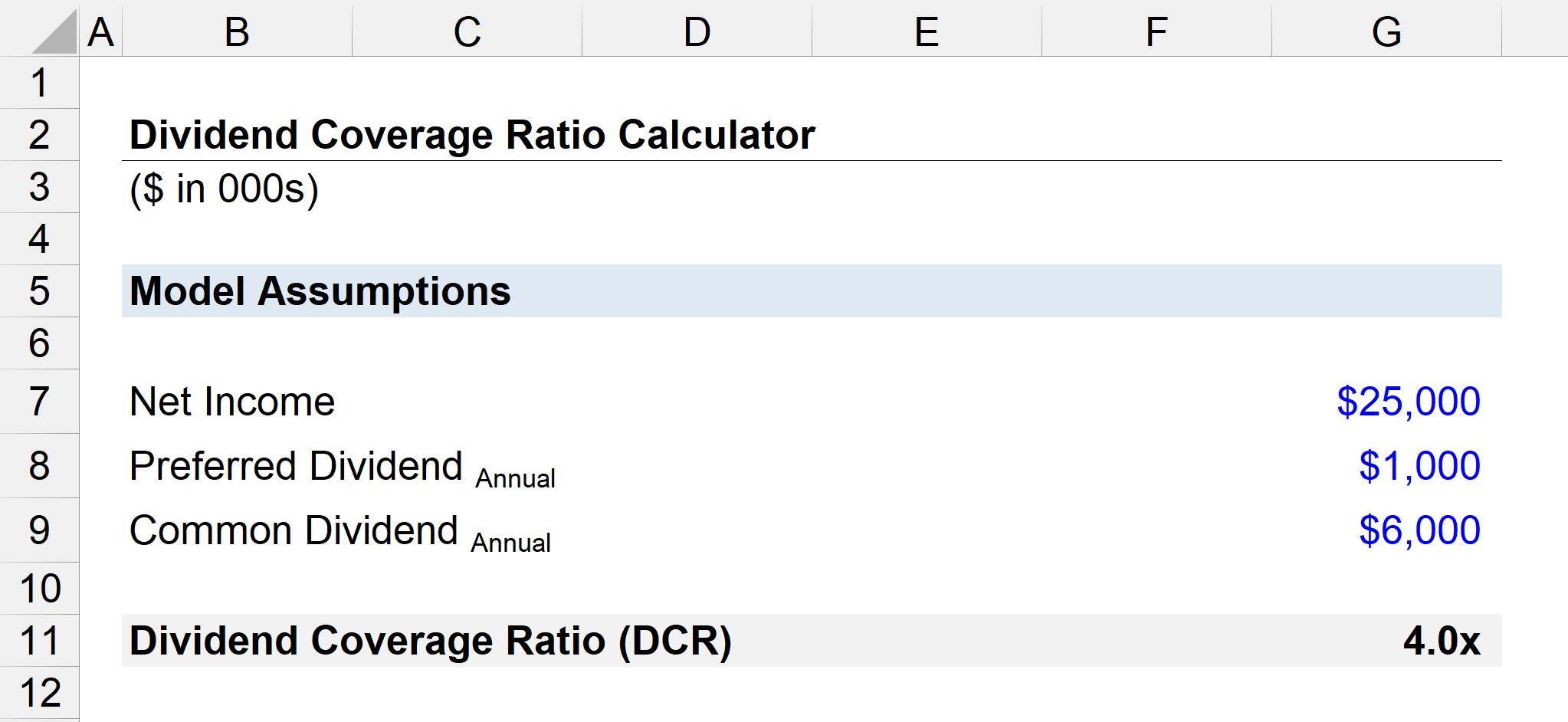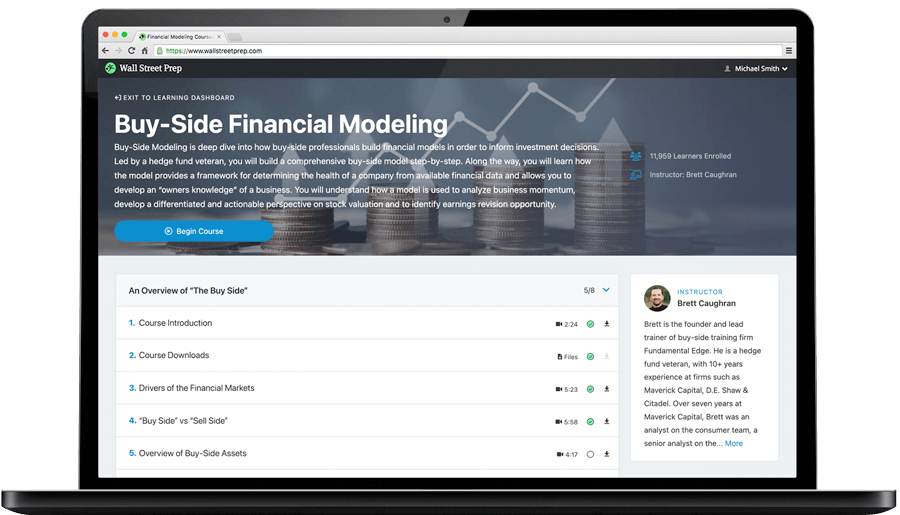Welcome to Wall Street Prep! Use code at checkout for 15% off.# Dividend Coverage Ratio

Guide to Understanding the Dividend Coverage Ratio (DCR)## How to Calculate Dividend Coverage Ratio (DCR)?

The dividend coverage ratio, or “dividend cover” for short, states how many times a company’s dividends can be paid using its net income.

The question answered by calculating the dividend cover metric is, “Is the company capable of continuing to pay out its dividend to shareholders into the foreseeable future?”

The dividend coverage ratio (DCR) enables shareholders to estimate the risk of a company being unable to issue its stated dividend.

Two common metrics tracked by shareholders are the dividend payout ratio and the dividend yield.

The dividend payout ratio measures the proportion of a company’s net income paid out as dividends, expressed as a percentage.

Dividend Payout Ratio (%) = Dividend Per Share (DPS) ÷ Earnings Per Share (EPS)

On the other hand, the dividend yield measures the dividend per share (DPS) relative to its latest closing share price, expressed in the form of a percentage.

Dividend Yield (%) = Dividend Per Share (DPS) ÷ Current Share Price

However, the dividend cover metric is usually used to determine the risk of the investor no longer receiving a dividend, which is conceptually similar to the interest coverage ratio for debt holders.

But unlike interest expense, a company is not obligated to pay out a dividend to shareholders, i.e. it cannot default on a discretionary payment to shareholders.

## Dividend Coverage Ratio Formula

To calculate the dividend coverage ratio (DCR) from the perspective of a common shareholder, the first step is to subtract the preferred dividend amount from net income.

Dividends to all equity holders, both common and preferred, are paid out of retained earnings, but common shareholders are placed below preferred shareholders in the capital structure.

Thus, common shareholders cannot be issued their dividend unless preferred shareholders are first compensated in full.

After net income is adjusted for preferred dividends, the next step is to divide by the dividend amount attributable to common shareholders.

Dividend Coverage Ratio = (Net IncomePreferred Dividend) ÷ Common Dividend

Conversely, the dividend cover can be calculated using the earnings per share (EPS) and dividend per share (DPS), but the numerator must be adjusted for the payout to preferred stockholders.

Another variation is to replace net income with cash flow from operations (CFO), which many view as a more conservative measure as it is less susceptible to earnings management.

## What is a Good Dividend Coverage Ratio? (DCR)

Since the dividend coverage ratio calculates the number of times that a company’s net earnings can meet its dividend amount, a higher ratio is “better.”

• DCR <1.0x → Net income is insufficient to pay the dividend
• DCR >1.0x → Net income is adequate to pay the dividend
• DCR >2.0x → Net income is more than sufficient to issue the dividend (2.0x)

Generally, a dividend coverage ratio (DCR) above 2.0x is perceived as the minimum “floor” before shareholders should be concerned regarding the sustainability of a company’s future dividends.

## Dividend Coverage Ratio Calculator

We’ll now move to a modeling exercise, which you can access by filling out the form below.#### Get the Excel Template!Submitting...

## Dividend Coverage Ratio Calculation Example

Suppose a company reported \$25 million in net income with a longstanding annual dividend of \$6 million announced to common shareholders.

“If the dividend paid to preferred stockholders was \$1 million, what is the dividend cover?”

After subtracting the preferred dividend from net income, we’re left with \$24 million of net income that could hypothetically be distributed to common shareholders.

With that said, the next step is to divide the leftover net income by the annual dividend to common shareholders to arrive at 4.0x as the dividend coverage ratio.

• Dividend Coverage Ratio = \$24 million ÷ \$6 million  = 4.0x

Given the 4.0x dividend coverage ratio, the company’s net income is sufficient to pay its annual dividend four times, so the common shareholders are unlikely to be concerned about an upcoming reduction in their dividend payments.Step-by-Step Online Course

### Learn Buy-Side (Hedge Fund) Modeling

Led by a former hedge fund PM (Maverick, Citadel, DE Shaw, Schonfeld), this program begins where financial modeling training ends — with a deep-dive into how buy-side analysts build financial models to make key investment decisions.